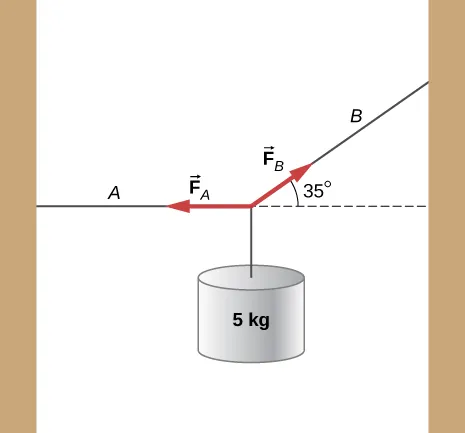University Physics Volume 1

# Challenge Problems

University Physics Volume 1Challenge Problems

### Challenge Problems

144.

A copper wire has a radius of $200μm200μm$ and a length of 5.0 m. The wire is placed under a tension of 3000 N and the wire stretches by a small amount. The wire is plucked and a pulse travels down the wire. What is the propagation speed of the pulse? (Assume the temperature does not change: $(ρ=8.96gcm3,Y=1.1×1011Nm).)(ρ=8.96gcm3,Y=1.1×1011Nm).)$

145.

A pulse moving along the x axis can be modeled as the wave function $y(x,t)=4.00me−(x+(2.00m/s)t1.00m)2.y(x,t)=4.00me−(x+(2.00m/s)t1.00m)2.$ (a)What are the direction and propagation speed of the pulse? (b) How far has the wave moved in 3.00 s? (c) Plot the pulse using a spreadsheet at time $t=0.00st=0.00s$ and $t=3.00st=3.00s$ to verify your answer in part (b).

146.

A string with a linear mass density of $μ=0.0085kg/mμ=0.0085kg/m$ is fixed at both ends. A 5.0-kg mass is hung from the string, as shown below. If a pulse is sent along section A, what is the wave speed in section A and the wave speed in section B?147.

Consider two wave functions $y1(x,t)=Asin(kx−ωt)y1(x,t)=Asin(kx−ωt)$ and $y2(x,t)=Asin(kx+ωt+ϕ)y2(x,t)=Asin(kx+ωt+ϕ)$. What is the wave function resulting from the interference of the two wave? (Hint: $sin(α±β)=sinαcosβ±cosαsinβsin(α±β)=sinαcosβ±cosαsinβ$ and $ϕ=ϕ2+ϕ2ϕ=ϕ2+ϕ2$.)

148.

The wave function that models a standing wave is given as $yR(x,t)=6.00cmsin(3.00m−1x+1.20rad)yR(x,t)=6.00cmsin(3.00m−1x+1.20rad)$ $cos(6.00s−1t+1.20rad)cos(6.00s−1t+1.20rad)$. What are two wave functions that interfere to form this wave function? Plot the two wave functions and the sum of the sum of the two wave functions at $t=1.00st=1.00s$ to verify your answer.

149.

Consider two wave functions $y1(x,t)=Asin(kx−ωt)y1(x,t)=Asin(kx−ωt)$ and $y2(x,t)=Asin(kx+ωt+ϕ)y2(x,t)=Asin(kx+ωt+ϕ)$. The resultant wave form when you add the two functions is $yR=2Asin(kx+ϕ2)cos(ωt+ϕ2).yR=2Asin(kx+ϕ2)cos(ωt+ϕ2).$ Consider the case where $A=0.03m−1,A=0.03m−1,$ $k=1.26m−1,k=1.26m−1,$ $ω=πs−1ω=πs−1$, and $ϕ=π10ϕ=π10$. (a) Where are the first three nodes of the standing wave function starting at zero and moving in the positive x direction? (b) Using a spreadsheet, plot the two wave functions and the resulting function at time $t=1.00st=1.00s$ to verify your answer.

Order a print copy

As an Amazon Associate we earn from qualifying purchases.